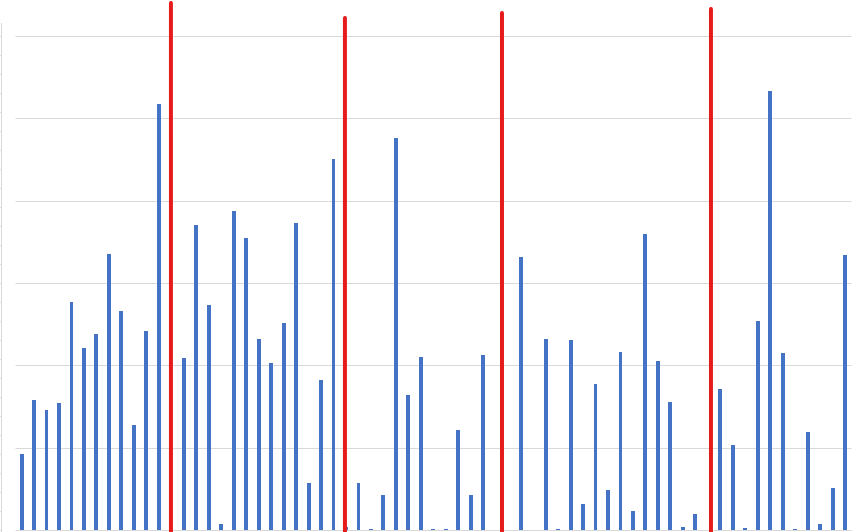# What are the Mapping Classification Methods?

When creating a map, you will be prompted to select a method. This refers to the method of classification. The four available options are Natural Breaks, Quantiles, Equal Intervals, and Standard Deviation.

## Natural Breaks

Natural breaks (also known as Jenks natural breaks or optimization) is a data classification method that identifies the natural groupings inherent in the data. As seen in the figure below represented by the red lines, natural break is identified by the “peaks” and “valleys” found in the data when formatted as a histogram. The method seeks to minimize the variance within each class while maximizing the variance between classes, optimizing the uniqueness of each class. Natural breaks are based on the principle that there are significant differences in the data distribution, which can be used to divide the data into meaningful classes. The figure below shows how the natural breaks method might segment data, given 4 breaks.### Pros:

• Minimize variance/differences for elements with the class, and maximize the variance/differences between classes.
• Highly accurate at finding trends within the data.

### Cons:

• Loses accuracy on data with a low variance; data that is too uniform.
• Can create widely varying number ranges.

### When should I use Natural Breaks?

Natural breaks is best used when you want to isolate groups in an otherwise uneven or seemingly random distributed set of data. Natural breaks will find clusters within that data and show where the highest levels of similarities and differences are found.

## Quantiles

Quantiles are a method of data classification that divides the data into equal portions based on the distribution of the data across the sample geography. For example, if the data is divided into four quantiles, each quantile contains a percentile equal to 25% of the geography's data. This method is useful when you want to create equal-sized classes and ensure that the classes are representative of the data distribution.

### Pros:

• Using the quantile classification method gives data classes at the extremes and middle the same number of values.
• Each class is equally represented on the map and the classes are easy to compute.
• Best when using ordinal data (data with a hierarchy but no specific numerical values, ie. “likelihood to participate in a behaviour”).

### Cons:

• Gaps can occur between the attribute values.
• Depending on the number of quantiles chosen, two identical values can end up in different groups.

### When should I use Quantiles?

Quantiles are best used when all possible categories have equal significance and need to be equally represented on the map. For example, if preference to shop at a specific store is being mapped across dissemination areas (DAs) with a max population of 100 and equal interval was used with 4 classes, each class would represent one quarter of the range (0 to 25, 26 to 50, 51 to 75, 76 to 100). However, if quantiles was used with 4 classes, the algorithm may skew lower and create classes of 0 to 10, 11 to 40, 41 to 70, 71 to 100. In this case, 25% of the DAs fall into each group, removing under-representation of the middle classes in the equal interval representation of the data.

## Equal Interval

Equal interval is a method of data classification that divides the range of the data into equal intervals of data instances, as opposed to quantiles which are divided into equal proportions. For example, if the data range is from 0 to 100, and you want to create four classes, each class would be 25 units, regardless of which percentile those units fall into. This method is useful when you want to create classes that have an equal range of values.

### Pros:

• Easily and quickly interpreted.
• Can highlight over- or under-representation in the data.

### Cons:

• Does not account for any characteristics of the data in regard to its value or distribution.

### When should I use Equal Interval?

Equal interval is the simplest representation of data and is best used for quick maps with a high-level look at the distribution of the data. For example, equal interval could be used for hierarchy of population, or spending habits, showing at a high level where the top, middle, and bottom population or spenders are. When a more in-depth review of these habits is needed, another method of classification can then be applied.

## Standard Deviation

Standard deviation is a statistical measure that indicates the amount of variation or dispersion in the data and is used to divide the data into classes based on how much they deviate from the mean value. For example, you could use standard deviation to create classes of data values that are within one, two, or three standard deviations of the mean. This method is similar to natural breaks, except each group is defined based on variance from the mean/average rather than the variance within and between each other.

### Pros:

• Reduces the impact of outliers.
• Highlights the main concentration of data.
• High accuracy on how data is distributed.

### Cons:

• May not provide the complete range of data, depending on the number of intervals chosen.
• Assumes data follows a normal distribution.

### When should I use Standard Deviation?

Standard deviation is best used when comparing data to an average. For example, standard deviation could show which areas are above, below, or equal to the average income. The intervals selected (between 0 and 1) will further break down how far these areas are from that average.

Overall, the choice of data classification method depends on the purpose of the analysis and the data being used. Different methods may lead to different class boundaries and affect the resulting analysis and visual representation.